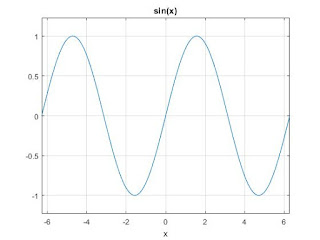World's most popular travel blog for travel bloggers.

# How Euclidean Coordinate System Differs from Homogeneous Coordinate system? What is the advantage of Homogeneous Coordinate system over Euclidean Coordinate System? Assume a polygon ABCDE having coordinates A(0,0), B(10,10), C(10,2), D(5,5), E(7,6) is subjected to the counter-clockwise rotation of 45° about an axis passing through the centroid of the polygon . Find the transformed coordinates of the polygon ABCDE. You should represent the transformation using Homogeneous Coordinate System

, ,

### How Euclidean Coordinate System Differs from Homogeneous Coordinate system?

Point in Euclidean plane can be written in many ways: either using Cartesian coordinate system, or polar coordinate system. That is same point pp can be written in two ways... If we are saying Euclidean plane, It simply means that we are giving some axioms and using theorem based on that axioms. But if we are saying Cartesian plane, it means that with euclidean axiom we are giving some method of representing of points.

This means: Euclidean Plane means we have only some set of axiom

Cartesian plane means Euclidean plane+ One fixed method of representing points.
The Cartesian system is Euclidean space with coordinates. The Cartesian Coordinate System unified geometry and algebra into one system of analytic geometry.

If you know MATLAB, A weak way of explaining it is:

``````clf;
ezplot('sin(x)'); %Euclidean
grid ON; %Cartesian``````
EuclideanEuclidean Sine Wave (No Coord System)

CartesianSine Wave in Cartesian Space (With Grid indicating coord system)

### ADVANTAGES OF HOMOGENEOUS COORDINATES

#### Representing all transformations as matrix multiplications

Two Dimensional coordinates  are represented using three-element column vectors, and Transformation operation is represented by 3 x 3 matrices.

which can be written in abbreviated form as

#### Capturing composite transformations conveniently

On the basis of the matrix product of the individual transformations we can set up a matrix for any sequence of transformation known as composite transformation matrix. For row-matrix representation we form composite transformations by multiplying matrices in order from left to right whereas in column-matrix representation we form composite transformations by multiplying matrices in order from right to left.

#### Representing points at infinity.

Homogeneous coordinates can be used to display a point at infinity. For example

In the above example the point at infinity is presented in the form of homogeneous coordinates. This is often needed when we want to represent a point at infinity in a certain direction. For instance, for finding the vanishing point in perspective projections we can transform the point at infinity in the given direction.

#### Post a Comment

Let us know your responses and feedback